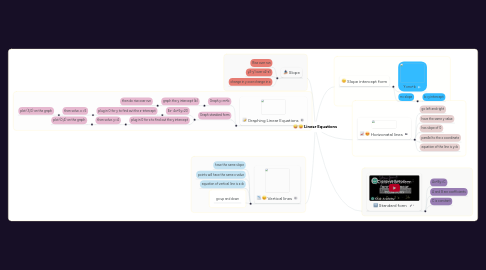# Linear EquationsDasia Harrigan
Get Started. It's FreeLinear Equations## 2. Slope intercept form

### 2.2. m=slope

2.2.1. b=y intercept

## 4. Graphing Linear Equations

### 4.1. Graph y=m+b

4.1.1. graph the y intercept (b)

4.1.1.1. then do rise over run

### 4.2. Graph standard form

4.2.1. Ex- 4x+5y=20

4.2.1.1. plug in 0 for y to find out the x-intercept

4.2.1.1.1. then solve. x =5

4.2.2. plug in 0 for x to find out the y intercept

4.2.2.1. then solve. y=4

4.2.2.1.1. plot (0,4) on the graph